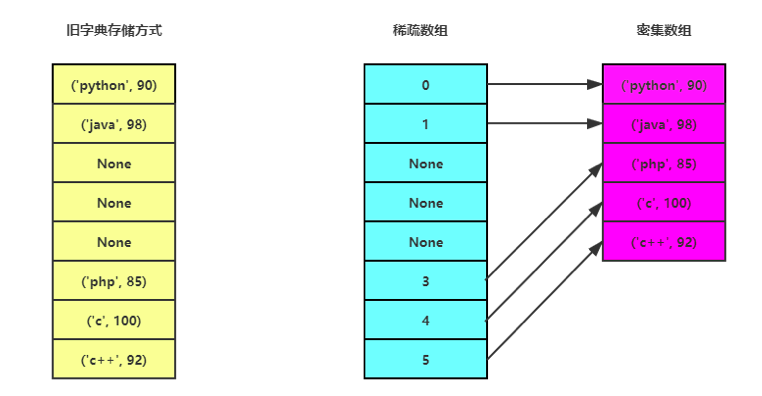# 掌握hashtable，深度理解python字典的数据结构

1. hash函数
2. hashtable数据结构
3. python字典顺序写入

## 1. hash函数

hash函数是一个统称，有许多种实现算法，例如MD5、SHA-1、SHA-2、NTLM等等。

1. 速度必须快，因为hash应用太广泛了，太基础了，速度慢是不可接受的
2. 映射的结果是确定的，同一个原始输入，经hash函数处理后得到的散列值必须相同，如果两个散列值不同，那么他们的原始输入也不相同
3. hash函数产生固定长度的值，这里的固定长度指的是存储散列值所占用的字节数相同

python的内置函数hash就是一个hash函数，它可以计算任意不可变对象的hash值

``````>>> hash('323')
-2887333350099739771
>>> hash(323)
323
>>> hash(4334.2323)
535647331039580398
>>> hash((1, 3))
3713081631933328131
``````

hash函数返回的是int类型数据，前面已经强调过，hash函数返回固定长度的值，这里的固定长度，指的是存储这个值所用的字节数，虽然这些int类型的数值大小不同，看起来长度不一致，但存储他们所用的字节数是相同的。对于自定义类，可以重写魔法方法__hash__来实现自己的hash函数。

``````>>> hash([1, 2, 3])
Traceback (most recent call last):
File "<stdin>", line 1, in <module>
TypeError: unhashable type: 'list'
``````

## 2. hashtable

### 2.1 链地址法实现hashtable

hashtable是一种可以存储键值对的数据结果。

hashtable的实现基础是数组，数组这种数据结构在内存层面是，是一片连续的空间，通过索引来存储和读取数据，效率自然是高。python里没有数组，因此，我用列表来实现一个hastable。

``````class PyHashTable():
def __init__(self, datas=None):
if datas is None:
self.length = 8
else:
self.length = len(datas)

self.buckets = [[] for i in range(self.length)]
self.init_buckets(datas)

def init_buckets(self, datas):
if datas is None:
return
for key, value in datas:
self.__setitem__(key, value)

def __getitem__(self, search_key):
hash_value = abs(hash(search_key))
index = hash_value % self.length
for key, value in self.buckets[index]:
if search_key == key:
return value

def __setitem__(self, key, value):
hash_value = abs(hash(key))
index = hash_value % self.length
self.buckets[index].append((key, value))

datas = [('python', 90), ('java', 98), ('php', 85), ('c', 100)]
hashtable = PyHashTable(datas)
print(hashtable['c'])   # 像使用字典一样

hashtable['c++'] = 92
print(hashtable['c++'])

print(hashtable.buckets)
``````

``````100
92
[
[],
[('python', 90), ('c++', 92)],
[('java', 98), ('c', 100)],
[('php', 85)]]
``````

PyHashTable，并不是一个完整的实现，一些功能并没有添加，比如删除某个key，但已经将hashtable最核心的结构和操作体现出来了，写入新的key-value对，通过key获取value，操作方式和字典是一样的。

### 2.2 解决冲突

hashtable解决冲突的方法常见的有两种：

1. 开放寻址
2. 链地址法

1. 新写入一个key2-value, kye2恰好被分配到n + k号，而此时n + k号桶被key1占据了，key2只好向下寻找空桶。
2. key1本应放到n号桶，而实际存放位置是n + k 号桶，根据key1查询value时，先到n号桶查询，n号桶里的key自然不等于key1， 于是继续向下寻找直到遇到一个空桶（表明key1不存在），key1在n + k号桶里，这样的寻找过程，要比较k次，如果大量的key都存在冲突，不论是写入还是查询，其性能都会有所下降。
3. 开放寻址可以避免空间的浪费，但是不同于链地址法，当buckets被装满时，就找不到一个空的桶可以使用了，这个时候怎么办呢？

2，3两个担忧，的确是个问题，但可以用同一个方法来解决，这个方法是扩容。python实现的hashtable，有2/3被装满时，就会进行扩容，扩容后，就不必担心没有空桶可用了，扩容也会减小k的数值，不至于出现性能下降。

### 2.3 开放寻址法实现hashtable

``````# program_dict = {
#     'python': 90,
#     'java': 98,
#     'php': 85,
#     'c': 100
# }
#
# print(program_dict)

class PyHashTable():
def __init__(self, datas=None):
if datas is None:
self.length = 8
else:
self.length = len(datas) * 2

self.buckets = [None for i in range(self.length)]
self.init_buckets(datas)

def init_buckets(self, datas):
if datas is None:
return
for key, value in datas:
self.__setitem__(key, value)

def __getitem__(self, search_key):
hash_value = abs(hash(search_key))
index = hash_value % self.length
while self.buckets[index] is not None:
if self.buckets[index] == search_key:
return self.buckets[index]
index = (index + 1) % self.length

return None

def __setitem__(self, key, value):
hash_value = abs(hash(key))
index = hash_value % self.length
while self.buckets[index] is not None:
index = (index + 1) % self.length

self.buckets[index] = (key, value)

datas = [('python', 90), ('java', 98), ('php', 85), ('c', 100)]
hashtable = PyHashTable(datas)
print(hashtable['c'])

hashtable['c++'] = 92
print(hashtable['c++'])

print(hashtable.buckets)
``````

``````100
92
[
('python', 90),
('java', 98),
None,
None,
None,
('php', 85),
('c', 100),
('c++', 92)
]
``````

``````    def __init__(self, datas=None):
if datas is None:
self.length = 8
else:
self.length = len(datas) * 2

self.buckets = [None for i in range(self.length)]
``````

``````    def __setitem__(self, key, value):
hash_value = abs(hash(key))
index = hash_value % self.length
while self.buckets[index] is not None:
index = (index + 1) % self.length

self.buckets[index] = (key, value)
``````

``````    def __getitem__(self, search_key):
hash_value = abs(hash(search_key))
index = hash_value % self.length
while self.buckets[index] is not None:
if self.buckets[index] == search_key:
return self.buckets[index]
index = (index + 1) % self.length

return None
``````

### 2.4 逻辑删除key

``````class HasDeleted():
def __getitem__(self, item):
return None

class PyHashTable():

def __delitem__(self, del_key):
hash_value = abs(hash(del_key))
index = hash_value % self.length
while self.buckets[index] is not None:
if self.buckets[index] == del_key:
self.buckets[index] = HasDeleted()
return True

index = (index + 1) % self.length

return False
``````

``````    def __setitem__(self, key, value):
hash_value = abs(hash(key))
index = hash_value % self.length
while not isinstance(self.buckets[index], (type(None), HasDeleted)):
index = (index + 1) % self.length

self.buckets[index] = (key, value)
``````

## 3. python的字典

``````program_dict = {
'python': 90,
'java': 98,
'php': 85,
'c': 100
}

print(program_dict)
``````

``````{
'python': 90,
'c': 100,
'php': 85,
'java': 98
}
``````

``````{
'python': 90,
'java': 98,
'php': 85,
'c': 100
}
``````QQ交流群: 211426309